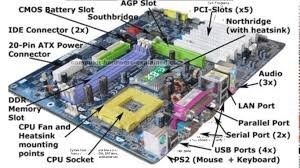# Central Processing Unit

Welcome to Class !!

In today’s Computer Science class, We will be learning about the Central Processing Unit. We hope you enjoy the class!CPU is the hardware part of a computer that carries out the instruction of a computer program by performing the basic arithmetic, logical and input/output operations of the system. CPU is the brain of the computer where most operations, calculations and processes take place.###### Two main components of a CPU

1. The arithmetic logic unit (ALU) – it performs arithmetic and logical operation. ALU carries out arithmetic operations on integer (whole number) and real (with a decimal point) operands.
2. The control unit (CU) – it extracts instruction from the computer memory, decodes and executes them and also transferring them to the ALU when necessary. The CU controls the fetching of instructions from the main memory and the subsequent execution of these instructions.

###### Control unit Functions
1. The control unit directs the entire computer system to carry out stored program instructions.
2. The control unit must communicate with both the arithmetic logic unit and main memory.
3. The control unit uses the instruction contained in the Instruction Register to decide which circuits need to be activated.
4. The control unit coordinates the activities of the other two units as well as all peripheral and auxiliary storage devices linked to the computer.
5. The control unit instructs the arithmetic logic unit which arithmetic operations or logical operations are to be performed.
6. The control unit is literally in control.

###### Functions of Arithmetic Logic Unit
1. The arithmetic logic unit executes arithmetic and logical operations.
2. Arithmetic operations include addition, subtraction, multiplication and division.
3. Logical operations compare numbers, letters and special characters.
4. Comparison operations test for three conditions:
• equal-to condition in which two values are the same
• less-than condition in which one value is smaller than the other
• greater-than condition in which one value is larger than the other
5. Relational operations (=, <, >) are used to describe the comparison operations used by the arithmetic logic unit.
6. The arithmetic logic unit performs logic functions such as AND, OR and NOT

We have come to the end of this class. We do hope you enjoyed the class?

Should you have any further question, feel free to ask in the comment section below and trust us to respond as soon as possible.

In our next class, we will be learning about the Memory Unit. We are very much eager to meet you there.

Are you a Parent? Share your quick opinion and win free 2-month Premium Subscription

Don`t copy text!